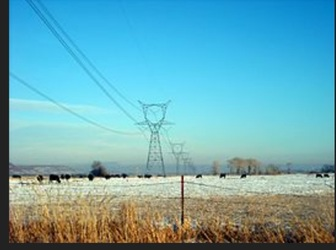AC circuit containing resistance onlyAC Circuit Containing Resistance Only

The pure resistive AC circuit contains only pure resistance of R ohms. There will be no effect of inductance and capacitance in this circuit. The alternate current and voltage move along both the directions as backwards and forwards. Therefore, current and voltage follow a shape of sine.

In a purely resistive circuit, the power is dissipated by the resistors and phase of both voltage and current remains the same. Which means that the voltage and current reaches a maximum value at the same time.Let the supply voltage be,

v = Vmsinωt ……(1)

The instantaneous value of current flowing through the given circuit is,

i = v / R = Vm / R sinωt ……(2)

From the equation (2), the value of current be maximum at t = 90°, so sinωt =1

Then, the instantaneous value of current will be,

i = Imsinωt ……(3)

So, by observing the equation (1) and (3), it is clear that there is no phase difference between the applied voltage and current flowing through the circuit. Meaning, phase angle between voltage and current is zero.

Hence, in a pure resistive ac circuit, the current is in phase with the voltage.

This can be expressed in a waveform as,